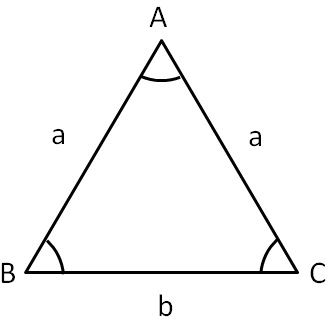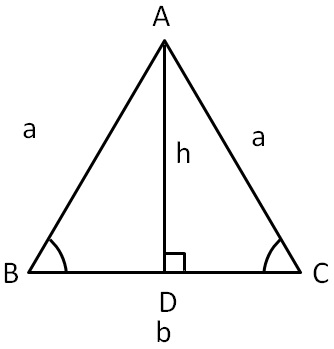Equilateral and Isoceles Triangle

Chapter 6 Class 7 Triangle and its Properties
Concept wise

Isosceles triangle is a triangle where

• Two sides are equal
• Angles opposite to equal sides are equalHere,

• a is the side which is equal, AB = AC
• b is the base, BC = b
• Angles opposite to equal sides are equal, ∠B = ∠C ( Check Proof )

Perimeter

Perimeter of isosceles triangle = Sum of all sides

= a + a + b

= 2a + b

Perimeter of isosceles triangle = 2a + bProperties of Isosceles triangle• Two sides are equal
• Angles opposite to equal sides are equal
• Altitude is the median

& D is the mid – point of BC

Learn in your speed, with individual attention - Teachoo Maths 1-on-1 Class